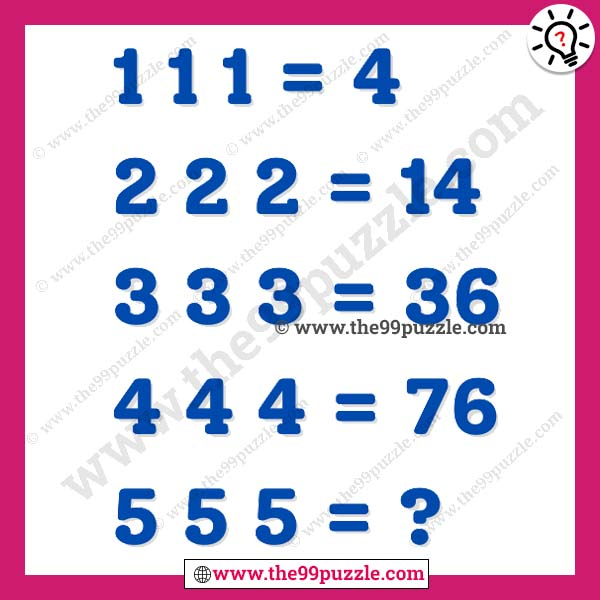# Reasoning mathematics puzzle with answer – Puzz282

Reasoning mathematics puzzle is very important for any competitive exam. Only genius people can solve these logical tricky puzzle tricks. If you consider yourself a genius then you must answer this question. Here you will find new number puzzles every day. Each puzzle will improve your math skills. These types of maths ask in every competitive exam. If you practice these math puzzles every day then it will be easier to crack any of your exams and you will be able to solve them in less time. There is a trick to this math puzzle. First, you have to find the tricks of this mathematics.

1 1 1 = 4

2 2 2 = 14

3 3 3 = 36

4 4 4 = 76

5 5 5 =?###### Explanation:

1 1 1 = (1×1×1)+(1+1+1) = 4

2 2 2 = (2×2×2)+(2+2+2) = 14

3 3 3 = (3×3×3)+(3+3+3) = 36

4 4 4 = (4×4×4)+(4+4+4) = 76

5 5 5 = (5×5×5)+(5+5+5) = 140